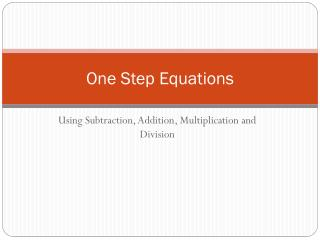# One Step Equations - PowerPoint PPT PresentationDownload PresentationOne Step Equations

One Step EquationsDownload Presentation## One Step Equations

- - - - - - - - - - - - - - - - - - - - - - - - - - - E N D - - - - - - - - - - - - - - - - - - - - - - - - - - -
##### Presentation Transcript

1. One Step Equations Using Subtraction, Addition, Multiplication and Division

2. How do we solve an equation??? • We have to isolate the variable. • To do this you have to get the variable with a coefficient of 1 alone on one side of the equation. • You can isolate a variable using the properties of equality and inverse operations. • An inverse operation undoes another operation.

3. Solving an Equation Using Subtraction X + 13 = 27 (You need to isolate x. Start by writing the equation.) X + 13 – 13 = 27 – 13 (Undo addition by subtracting the same number from each side.) What Property is this? X = 14 (Simplify each side of the equation.) Next…You must ALWAYS check your work!!!

4. Checking your work. • Always check your work and you will make sure you have the correct answer. X + 13 = 27 (Start with the original problem.) 14 + 13 = 27 (Plug in what you found the variable to be.) 27 = 27 (This checks out!!)

6. Solving an Equation Using Addition -7 = b – 3 (Isolate the variable.) -7 + 3 = b – 3 + 3 (Undo Subtraction by adding the same number to both sides. What Property are you using?) -4 = b (Simplify.) Now you need to check your work. Like we did before.

9. Solving an Equation using Division 4x = 6.4 (Isolate the variable) (Undo multiplication by dividing the same number from both sides. What Property are you using?) (Simplify each side of the equation.) Check your answer now.